Skip to main content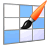# iRubric: Four Variable Matrix Project rubric

 find rubric(draft)           test run   apply to...   delete.rubinfoitem { padding:5px; white-space: nowrap; } Four Variable Matrix Project  Solve your four variable system using the the following methods: Elimination, Reduced Row Echelon, Cramer's Rule, Inverses Your work will be graded on accuracy, and organization. You will also write a paragraph about which method you liked the most and why and which one you dislike the most and why. Rubric Code: M224896 Draft Public Rubric Subject: Math   Type: Assessment   Grade Levels: 9-12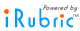Four Variable System Highly Proficient 8 pts Proficient 6 pts Partially Proficient 4 pts Minimally Proficient 2 pts Did Not Attempt 0 pts Elimination Highly Proficient Student solves the system following all steps to find a correct solution. Student can follow the steps correctly and steps are easy to follow, calculations are correct. Proficient Student completes the problem following most steps to find a correct solution. Student can follow the steps correctly and steps are easy to follow, calculations have only minor errors. Partially Proficient Student partially completes the problem or the wrong method is used to find a solution. Student can follow some of the steps to solve the problem but errors in calculation cause the answer to be incorrect. Minimally Proficient Student has minimal understanding of how to solve the matrices using the specified method, or they do not complete the problem. Did Not Attempt Student did not attempt the specified method. Reduced Row Echelon Highly Proficient Student solves the matrix following all steps to find a correct solution. Student can follow the steps correctly and steps are easy to follow, calculations are correct. Proficient Student completes the problem following most steps to find a correct solution. Student can follow the steps correctly and steps are easy to follow, calculations have only minor errors. Partially Proficient Student partially completes the problem or the wrong method is used to find a solution. Student can follow some of the steps to solve the problem but errors in calculation cause the answer to be incorrect. Minimally Proficient Student has minimal understanding of how to solve the matrices using the specified method, or they do not complete the problem. Did Not Attempt Student did not attempt the specified method. Cramer's Rule Highly Proficient Student solves the system following all steps for Cramer's Rule to find a correct solution. Student can follow the steps correctly and steps are easy to follow, calculations are correct. Proficient Student completes the problem following most steps to find a correct solution. Student can follow the steps correctly and steps are easy to follow, calculations have only minor errors. Partially Proficient Student partially completes the problem or the wrong method is used to find a solution. Student can follow some of the steps to solve the problem but errors in calculation cause the answer to be incorrect. Minimally Proficient Student has minimal understanding of how to solve the matrices using the specified method, or they do not complete the problem. Did Not Attempt Student did not attempt the specified method. Inverses Highly Proficient Student solves the system by using inverses correctly to find a correct solution. Student can follow the steps correctly and steps are easy to follow, calculations are correct. Proficient Student completes the problem following most steps to find a correct solution. Student can follow the steps correctly and steps are easy to follow, calculations have only minor errors. Partially Proficient Student partially completes the problem or the wrong method is used to find a solution. Student can follow some of the steps to solve the problem but errors in calculation cause the answer to be incorrect. Minimally Proficient Student has minimal understanding of how to solve the matrices using the specified method, or they do not complete the problem. Did Not Attempt Student did not attempt the specified method. Enter Title Highly Proficient 4 pts Proficient 3 pts Partially Proficient 2 pts Minimally Proficient 1 pts Did Not Attempt 0 pts Determinant Highly Proficient Student thoroughly and accurately explains how to find a 4x4 determinant or uses an example to show and label each step. A website is included as to where information was obtained. Proficient Student adequately explains how to find a 4x4 determinant or uses an example to show and label each step. A website is included as to where information was obtained. Partially Proficient Student explanation on how to find a 4x4 determinant or example used to show each step was not thorough or was not accurate. A website is included as to where information was obtained. Minimally Proficient Student explanation on how to find a 4x4 determinant or example used to show each step was not thorough or was not precise. A website is not included as to where information was obtained. Did Not Attempt The student omits this part of the project. Reflection Highly Proficient Student thoroughly explains what method they liked and dislike and why. Their thoughts are logical and there paragraph flows to the reader. Proficient Student explanation is adequate but not much reflection is shown in their writing. Their thoughts are logical and the paragraph flows to the reader. Partially Proficient Student provides minimal explanation but their reasoning was not fully developed in their paragraph. Minimally Proficient Student does not put much thought into their writing and has no concrete reason for their likes/dislikes. Did Not Attempt The student omits this part of the project. Neatness Highly Proficient Student's work is exceptionally neat and organized. Everything is labeled. Student could use colors to help with the flow of their work Proficient Student's work is neat and organized. Everything is labeled. Partially Proficient Student's work is neat and organized. Almost everything is labeled. Minimally Proficient Student's work does not logically flow and is not labeled. Did Not Attempt Student's work is extremely unorganized and writing is fairly illegible.

Subjects:

Types:

Do more with this rubric:Preview this rubric.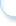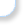Modify this rubric.Make a copy of this rubric and begin editing the copy.Show a printable version of this rubric.Add this rubric to multiple categories.Bookmark this rubric for future reference.Assess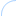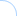This rubric is still in draft mode and cannot be scored. Please change the rubric status to ready to use.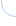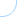ShareLink, embed, and showcase your rubrics on your website.Email this rubric to a friend.Discuss this rubric with other members.Do more with rubrics than ever imagined possible. Only with iRubrictm.About Education Sites & Communities My Account Support Enterprise Copyright © 2021 Reazon Systems, Inc.  All rights reserved.
n17